# Comparison theorem

in the theory of differential equations

A theorem that asserts the presence of a specific property of solutions of a differential equation (or system of differential equations) under the assumption that an auxiliary equation or inequality (system of differential equations or inequalities) possesses a certain property.

## Examples of comparison theorems.

1) Sturm's theorem: Any non-trivial solution of the equation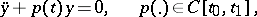vanishes on the segment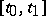at mosttimes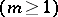if the equation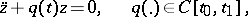possesses this property andwhen(see ).

2) A differential inequality: The solution of the problem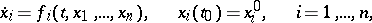is component-wise non-negative when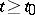if the solution of the problempossesses this property and if the inequalities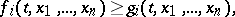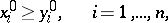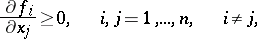are fulfilled (see ).

For other examples of comparison theorems, including the Chaplygin theorem, see Differential inequality. For comparison theorems for partial differential equations see, for example, .

One rich source for obtaining comparison theorems is the Lyapunov comparison principle with a vector function (see ). The idea of the comparison principle is as follows. Let a system of differential equations(1)

and vector functions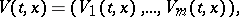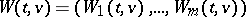be given, where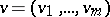. For any solution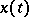of the system (1), the function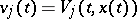,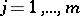, satisfies the equation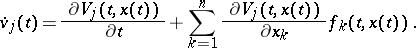Therefore, if the inequalities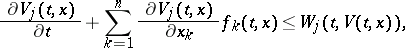(2)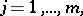are fulfilled, then on the basis of the properties of the system of differential inequalities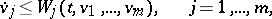(3)

something can be said about the behaviour of the functions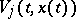that are solutions of the system (3). Knowing the behaviour of the functionson every solutionof the system (1), in turn, enables one to state assertions on the properties of the solutions of the system (1).

For example, let the vector functionsand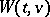satisfy the inequalities (2) and for any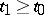,, let a numberexist such that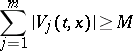for all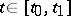,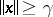. Furthermore, let every solution of the system of inequalities (3) be defined on. Every solution of the system (1) is then also defined on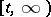.

A large number of interesting statements have been obtained on the basis of the comparison principle in the theory of the stability of motion (see ). The Lyapunov comparison principle with a vector function is successfully used for abstract differential equations, differential equations with distributed argument and differential inclusions (cf. Differential equation, abstract; Differential equations, ordinary, with distributed arguments; Differential inclusion). In particular, for a differential inclusion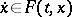,, where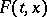is a set independent on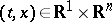, the role of the inequalities (2) is played by the inequalitiesA large number of comparison theorems are given in .